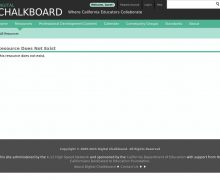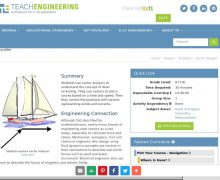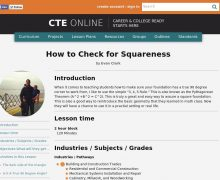• # Math: Geometry

Browse All 10 ResourcesIn this activity, students explore the importance of charts to navigation on bodies of water. Using one worksheet, students learn to read the major map features found on a real nau...Students will see an animated presentation of the proof of the Pythagorean Theorem. This animated PowerPoint presentation uses shearing and the invariance of the area of triangles ...Students use vector analysis to understand the concept of dead reckoning. They use vectors to plot a course based on a time and speed. Then they correct the positions with vectors ...These 20 images give students practice measuring the height and width of various pictures with paperclips. Ready to print, each sheet contains a spot for students to write in their...When it comes to teaching students how to make sure your foundation has a true 90 degree corner to work from, I like to use the simple "3, 4, 5 Rule." This is also known as the Pyt...In this activity, students explore the importance of charts to navigation on bodies of water. Using one worksheet, students learn to read the major map features found on a real nau...Students will see an animated presentation of the proof of the Pythagorean Theorem. This animated PowerPoint presentation uses shearing and the invariance of the area of triangles ...Students use vector analysis to understand the concept of dead reckoning. They use vectors to plot a course based on a time and speed. Then they correct the positions with vectors ...These 20 images give students practice measuring the height and width of various pictures with paperclips. Ready to print, each sheet contains a spot for students to write in their...When it comes to teaching students how to make sure your foundation has a true 90 degree corner to work from, I like to use the simple "3, 4, 5 Rule." This is also known as the Pyt...